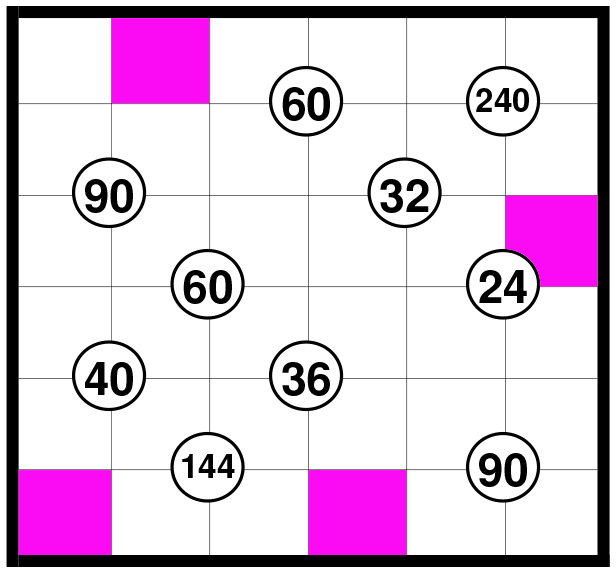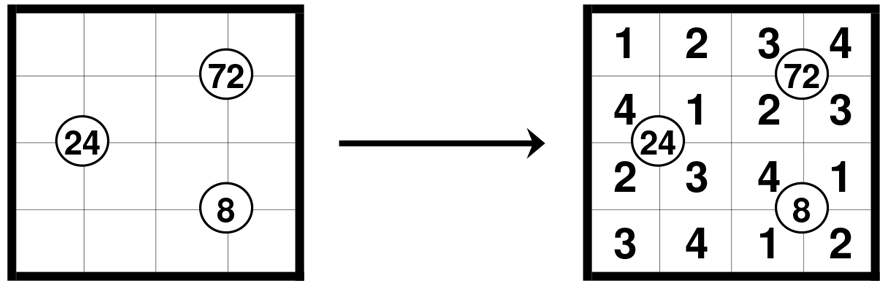# Product puzzle

Write a single positive digit $(\leq 6)$ into each empty square in the figure below, such that every number $(1, 2, 3, 4, 5, 6)$ appears in each row and in each column. The number in a circle shows the product of the four numbers around it.

If you are finished, find the minimum value of the product of the numbers in the pink squares!Note: Here is a sample, with digits $1, 2, 3, 4$:×